## Body mass index modifies the risk of cardiovascular death in proteinuric chronic kidney disease.

RP. Obermayr(1), C. Temml(2), G. Gutjahr(3), A. Kainz(4), R. Klauser-Braun(1), R. Függer(5), R. Oberbauer(4)

1- 3rd Medical Department (Division of Nephrology, Diabetes, Hypertension), Donauspital, Sozialmedizinisches Zentrum Ost der Stadt Wien, Austria

2- Department of Health Prevention, Vienna, Austria

3- Institute of Medical Statistics, Medical University Vienna, Austria

4- Department of Nephrology, KH Elisabethinen, Linz, and Medical University Vienna, & Austrian Dialysis and Transplant Registry, Austria

5- Department of Surgery, KH Elisabethinen, Linz, Austria

// Tables
// Figures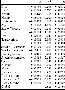Webtable 1:

Tests for proportional hazards assumption concerning cardiovascular mortality.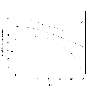Webfigure 1:

Scaled Schoenfeld residuals for the factor smoke concerning cardiovascular mortality.

Legend: Under the proportional hazards assumption, the residuals are constant over time. For the factor smoke, residuals are decreasing. Therefore a time dependent term was used for the factor smoke in the final model.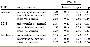Webtable 2:

Effect contrasts for cardiovascular death (alternative model 1)

Legend: To validate the model of the main text (table 3a) we fitted three additional models: In alternative model 1, variables were included as confounders in a multivariable Cox model if the change in the Chi-Square statistics of the BMI*GFR interaction term exceeded 10%. Contrasts were calculated identically to Table 3a of the main paper. Wald test for the effect size of the BMI*GFR interaction term: P = 0.0001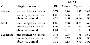Webtable 3:

Effect contrasts for cardiovascular death (alternative model 2)

Legend: To validate the model of the main text (table 3a) we fitted three additional models: For alternative model 2 a propensity score was created by logistic regression of cardiovascular death on all potential confounders. Alternative model 2 uses this propensity score in a Cox regression model stratified by quintiles of the propensity score. Contrasts were calculated identically to Table 3a of the main paper. Wald test for the effect size of the BMI*GFR interaction term: P = 0.0070.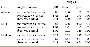Webtable 4:

Effect contrasts for cardiovascular death (alternative model 3)

Legend: To validate the model of the main text (table 3a) we fitted three additional models: For alternative model 2 a propensity score was created by logistic regression of cardiovascular death on all potential confounders. Alternative model 3 uses this propensity score as time dependent covariable in a Cox regression model. Contrasts were calculated identically to Table 3a of the main paper. Wald test for the effect size of the BMI*GFR interaction term: P = 0.0001.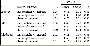Webtable 5:

Effect contrasts for cancer death (alternative model 1)

Legend: To validate the model of the main text (table 3a) we fitted three additional models: In alternative model 1, variables were included as confounders in a multivariable Cox model if the change in the Chi-Square statistics of the BMI*GFR interaction term exceeded 10%. Contrasts were calculated identically to Table 3a of the main paper. Wald test for the effect size of the BMI*GFR interaction term: P = 0.0808.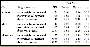Webtable 6:

Effect contrasts for cancer death (alternative model 2)

Legend: To validate the model of the main text (table 3a) we fitted three additional models: For alternative model 2 a propensity score was created by logistic regression of cardiovascular death on all potential confounders. Alternative model 2 uses this propensity score in a Cox regression model stratified by quintiles of the propensity score. Contrasts were calculated identically to Table 3a of the main paper. Wald test for the effect size of the BMI*GFR interaction term: P = 0.2609.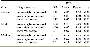Webtable 7:

Effect contrasts for cancer death (alternative model 3)

Legend: To validate the model of the main text (table 3a) we fitted three additional models: For alternative model 2 a propensity score was created by logistic regression of cardiovascular death on all potential confounders. Alternative model 3 uses this propensity score as time dependent covariable in a Cox regression model. Contrasts were calculated identically to Table 3a of the main paper. Wald test for the effect size of the BMI*GFR interaction term: P = 0.0829.

SCIENTIFIC COLLABORATIONS